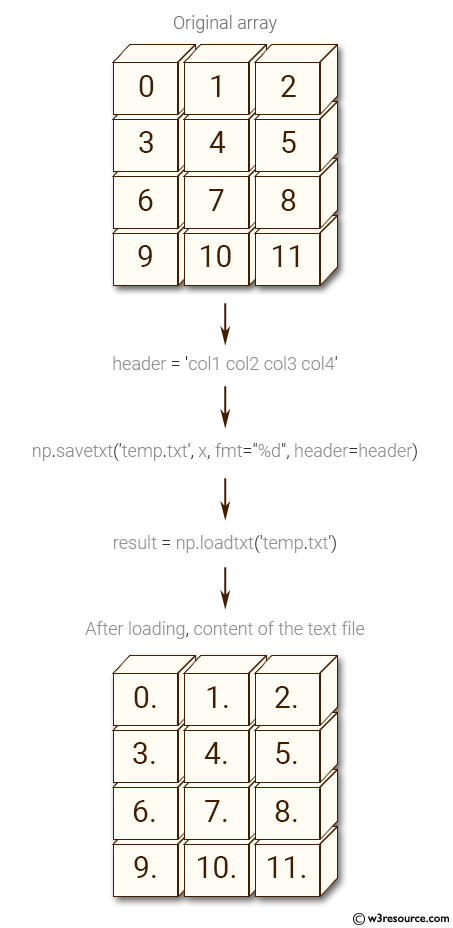﻿ NumPy: Save a given array to a text file and load it - w3resource

# NumPy: Save a given array to a text file and load it

## NumPy: Basic Exercise-37 with Solution

Write a NumPy program to save a given array to a text file and load it.

Sample Solution :

Python Code :

``````import numpy as np
import os
x = np.arange(12).reshape(4, 3)
print("Original array:")
print(x)
print(result)
``````

Sample Output:

```Original array:
[[ 0  1  2]
[ 3  4  5]
[ 6  7  8]
[ 9 10 11]]
[[  0.   1.   2.]
[  3.   4.   5.]
[  6.   7.   8.]
[  9.  10.  11.]]
```

Pictorial Presentation:Python Code Editor:

Have another way to solve this solution? Contribute your code (and comments) through Disqus.

What is the difficulty level of this exercise?

﻿

Inviting useful, relevant, well-written and unique guest posts